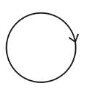# Consider a circular coil of wire carrying constant current I

Question:

Consider a circular coil of wire carrying constant current $I$, forming a magnetic dipole. The magnetic flux through an infinite plane that contains the circular coil and excluding the circular coil area is given by $\phi_{i}$.The magnetic flux through the area of the circular coil area is given by $\phi_{0}$. Which of the following option is correct?

1. (1) $\phi_{i}=\phi_{0}$

2. (2) $\phi_{i}>\phi_{0}$

3. (3) $\phi_{i}<\phi_{0}$

4. (4) $\phi_{i}=-\phi_{0}$

Correct Option: , 4

Solution:

(4) As magnetic field lines form close loop, hence every magnetic field line creating magnetic flux through the inner region $\left(\phi_{i}\right)$ must be passing through the outer region. Since flux in two regions are in opposite region.$\therefore \phi_{i}=-\phi_{0}$